# NCERT Solutions class-11 Maths Exercise 2.2## myCBSEguide App

CBSE, NCERT, JEE Main, NEET-UG, NDA, Exam Papers, Question Bank, NCERT Solutions, Exemplars, Revision Notes, Free Videos, MCQ Tests & more.

Exercise 2.2

1. Let A = {1, 2, 3, ……., 14}. Define a relation R from A to A by R =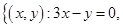where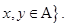Write down its domain co-domain and range.

Ans. Given: A = {1, 2, 3, ……….., 14}

The ordered pairs which satisfy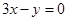are (1, 3), (2, 6), (3, 9) and (4, 12).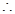R = {(1, 3), (2, 6), (3, 9), (4, 12)}

Domain = {1, 2, 3, 4}

Range = {3, 6, 9, 12}

Co-domain = {1, 2, 3, ……….., 14}

2. Define a relation R on the set N of natural numbers R =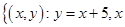is a natural number less than 4: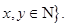Depict this relationship using roster form. Write down the domain and the range.

Ans. Given: R =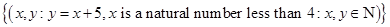Putting= 1, 2, 3 in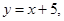we get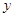= 6, 7, 8R = {(1, 6), (2, 7), (3, 8)}

Domain = {1, 2, 3}

Range = {6, 7, 8}

3. A = {1, 2, 3 5} and B = {4, 6, 9}. Define a relation R from A to B by R =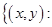the difference betweenandis odd: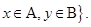Write R in roster form.

Ans. Given: A = {1, 2, 3, 5} and B = {4, 6, 9},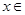A,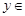B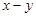= (1 – 4), (1 – 6), (1 – 9), (2 – 4), (2 – 6), (2 – 9), (3 – 4), (3 – 6) (3 – 9),

(5 – 4), (5 – 6), (5 – 9)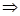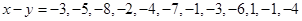R = {(1, 4), (1, 6), (2, 9), (3, 4), (3, 6) (5, 4), (5, 6)}

4. Figure shows a relationship between the sets P and Q. Write this relation:

(i) in set-builder form

(ii) roster form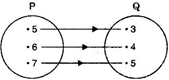What is its domain and range?

Ans. (i) Relation R in set-builder form is R =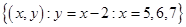(ii) Relation R in roster form is R = {(5 3), (6, 4), (7, 5)

Domain = {5, 6, 7}

Range = {3, 4, 5}

5. Let A = {1, 2, 3, 4, 6}. Let R be the relation on A defined by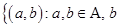is exactly divisible by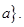(i) Write R in roster form.

(ii) Find the domain of R.

(iii) Find the range of R.

Ans. Given: A = {1, 2, 3, 4, 6}

A set of ordered pairs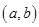where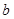is exactly divisible by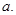(i) R = {(1, 1), (1, 2), (1, 3), (1, 4), (1, 6), (2, 2), (2, 4), (2, 6), (3, 3), (3, 6), (4, 4), (4, 6)}

(ii) Domain of R = {1, 2, 3, 4, 6}

(iii) Range of R = {1, 2, 3, 4, 6}

6. Determine the domain and range of the relation R defined by

R =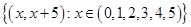Ans. Given: R ==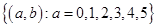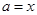and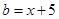Putting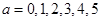we get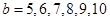Domain of R = {0, 1, 2, 3, 4 5}

Range of R = {0, 1, 2, 3, 4 5}

7. Write the relation R =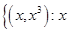is a prime number less than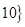in roster form.

Ans. Given: R =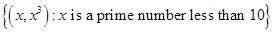Putting= 2, 3, 5, 7

R = {(2, 8), (3, 27), 5, 125), (7, 343)}

8. Let A =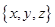and B = {1, 2}. Find the number of relations from A to B.

Ans. Given: A =and B = {1, 2}

Number of elements in set A = 3 and Number of elements in set B = 2Number of subsets of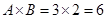Number of relations from A to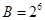.

9. Let R be the relation on Z defined by R =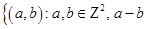is an integer}. Find the domain and range of R.

Ans. Given: R =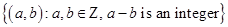=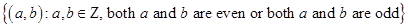=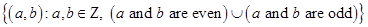Domain of R = Z

Range of R = Z